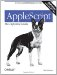# Section 9.4. Parameters

### 9.4. Parameters

One of the key features of a handler is that it can accept parameters . A parameter is a value passed to the handler as it is called. The handler can see these values, and can react to them. Thus, the handler can do different things depending on the values actually passed as parameters on each occasion when the handler is called.

For example, here's a definition of a handler that takes two parameters, and a call to that handler:

` on subtract(x, y)     return x - y end subtract subtract(3, 2) -- 1`

In the last line, the handler is called with the two parameters it requires. The value 3 is passed as the first parameter; the value 2 is passed as the second parameter. In the handler definition, names that effectively designate variables belonging to the handler have been declared. When the handler is called, and before it actually starts executing, these names are paired with the parameters that were passed, and the corresponding values are assigned. Thus, when add( ) is called on this occasion, it is as if it had a variable x which has been initialized to 3, and a variable y which has been initialized to 2. Thus, the result is 1. But the result would have been different if the parameter values passed in the last line had been 10 and 8. That's the point of parameters.

The names of the parameters within the handler, such as x and y in this example, designate variables local to the handler. The meaning and significance of this is explained in Chapter 10, but the key fact is that the names of the parameters in the handler definition have nothing to do with the names of the values passed as parameters. Consider this example:

` on subtract(x, y)     return x - y end subtract set x to 2 set y to 3 subtract(y, x) -- 1`

The handler subtracts y from x. If these were the same as the y and x at the top level of the script, you'd expect the result to be negative (we'd be subtracting 3 from 2). But in the handler call, the names are not what mattersthe values are. The value 3 is passed as the first parameter and becomes x within the handler. The value 2 is passed as the second parameter and becomes y within the handler.AppleScript: The Definitive Guide, 2nd Edition
ISBN: 0596102119
EAN: 2147483647
Year: 2006
Pages: 267
Authors: Matt Neuburg

Similar book on Amazon

flylib.com © 2008-2017.
If you may any questions please contact us: flylib@qtcs.net